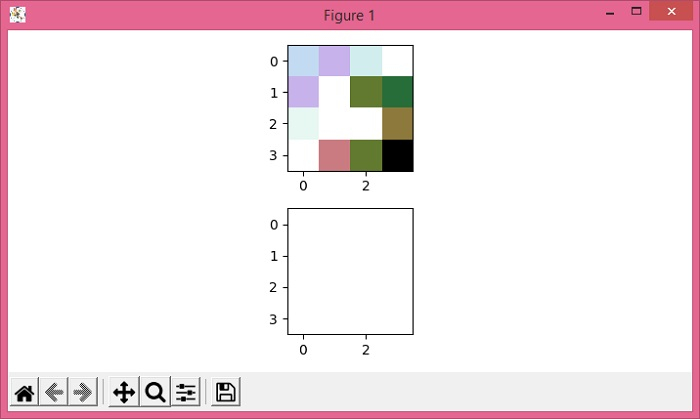# Gaussian filtering an image with NaN in Python Matplotlib

Gaussian filtering an image with NaN values makes all the values of a matrix NaN, which produces an NaN valued matrix.

## Steps

• Create a figure and a set of subplots.
• Create a matrix with NaN value in that matrix.
• Display the data as an image, i.e., on a 2D regular raster, data.
• Apply Gaussian filter on the data.
• Display the data as an image, i.e., on a 2D regular raster, gaussian_filter_data.
• To display the figure, use show() method.

## Example

import numpy as np
from matplotlib import pyplot as plt
from scipy.ndimage import gaussian_filter
plt.rcParams["figure.figsize"] = [7.00, 3.50]
plt.rcParams["figure.autolayout"] = True
fig, axes = plt.subplots(2)
data = np.array([[1., 1.2, 0.89, np.nan],
[1.2, np.nan, 1.89, 2.09],
[.78, .67, np.nan, 1.78],
[np.nan, 1.56, 1.89, 2.78]])
axes.imshow(data, cmap="cubehelix_r")
gaussian_filter_data = gaussian_filter(data, sigma=1)
axes.imshow(gaussian_filter_data, cmap="cubehelix_r")
plt.show()

## Output